# OmnesAudio

Omnes Audio develops speaker for car hifi, multimedia and home stereo systems.

## Tweeter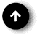open a bigger photo

## OmnesAudio AMT 21 S BP 1.1

order no. BP-amt-21sbp11

EUR 219,00excl. VAT: € 184.03 / \$ 206.12

air motion transformer.open a bigger photo

## OmnesAudio AMT 25 S BP 2.1

order no. BP-amt-25sbp21

EUR 329,00excl. VAT: € 276.47 / \$ 309.65

air motion transformer.

• power handling (continuous/programme) = 25 W
• frequency range = 1100-32000 Hz
• impedance R = 7 Ohm
• sound pressure level SPL = 91 dB (2,83V; 1m)

## Full Rangerecommended cabinet 1:
closed cabinet with 0,71 L volume
from 163/103 Hz (-3dB/-8dB)

recommended cabinet 2:
2,9 L volume bass reflex cabinet
with HP35 reflex tube, 10 cm long.
from 76/64 Hz (-3dB/-8dB).

## OmnesAudio BB 3.01

order no. BP-BB301

EUR 34,90excl. VAT: € 29.33 / \$ 32.85

8 cm full range driver with paper cone. The magnet system is based on Neodymium technology.

• power handling (continuous/programme) = 15/40 W
• frequency range = 100-20000 Hz
• resonance frequency fs = 97 Hz
• impedance R = 4 Ohm
• sound pressure level SPL = 86 dB (2,83V; 1m)
• DC resistance Re = 3,5 Ohm
• force factor BL = 2,89 N/A
• voice coil inductance L = 0,07 mH
• effective piston radiating area Sd = 31,1 cm2
• effective mechanical mass incl. air load mms = 2,33 g
• equivalent volume of compliance Vas = 1,56 l
• total Q factor Qts = 0,46 (Qms=2,52, Qes=0,56)
• mounting diameter d = 74 mm
• overall diameter d = 93 mm
• mounting depth (not countersunk) t = 45 mm
PDF data sheet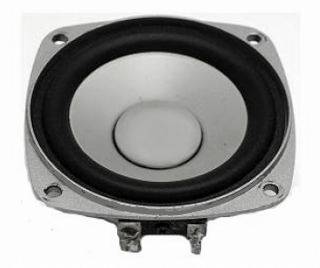recommended cabinet 1:
closed cabinet with 0,88 L volume
from 149/94 Hz (-3dB/-8dB)

recommended cabinet 2:
3,6 L volume bass reflex cabinet
with HP35 reflex tube, 10 cm long.
from 69/58 Hz (-3dB/-8dB).

## OmnesAudio BB 3.AL

order no. BP-BB3al

EUR 28,50excl. VAT: € 23.95 / \$ 26.82

8 cm full range driver with aluminium cone. The magnet system is based on Neodymium technology.

• frequency range = 80-20000 Hz
• resonance frequency fs = 94 Hz
• impedance R = 6 Ohm
• sound pressure level SPL = 83 dB (2,83V; 1m)
• DC resistance Re = 4,7 Ohm
• force factor BL = 3,3 N/A
• voice coil inductance L = 0,07 mH
• effective piston radiating area Sd = 29,2 cm2
• effective mechanical mass incl. air load mms = 2,1 g
• equivalent volume of compliance Vas = 1,6 l
• total Q factor Qts = 0,5 (Qms=6,2, Qes=0,54)
• voice coil diameter = 25 mm
• maximum peak linear excursion vibration xlin = +/- 1 mm
• mounting diameter d = 70 mm
• overall diameter d = 80 mm
• mounting depth (not countersunk) t = 39 mm
PDF data sheet

## Coaxial speaker## OmnesAudio CX 3.0

order no. BP-CX30

EUR 44,95excl. VAT: € 37.77 / \$ 42.31

95 x 95 cm coax driver with aluminium cone.

• frequency range = 200-20000 Hz
• resonance frequency fs = 173,51 Hz
• impedance R = 8 Ohm
• sound pressure level SPL = 84 dB (2,83V; 1m)
• DC resistance Re = 6 Ohm
• force factor BL = 4,96 N/A
• voice coil inductance L = 0,17 mH
• effective piston radiating area Sd = 48 cm2
• effective mechanical mass incl. air load mms = 3,6 g
• equivalent volume of compliance Vas = 0,74 l
• total Q factor Qts = 0,64 (Qms=2,94, Qes=0,81)
• voice coil diameter = 30 mm
• mounting diameter d = 75 mm
• overall diameter d = 95 x 95 mm
• mounting depth (not countersunk) t = 59 mm
PDF data sheet

## Woofer## no longer soldopen a bigger photo

## OmnesAudio T27

order no. BP-t27- not available

25 mm dome tweeter with fabric dome.

• frequency range = 1000-30000 Hz
• resonance frequency fs = 650 Hz
• impedance R = 4 Ohm
• sound pressure level SPL = 93,5 dB (2,83V; 1m)
• DC resistance Re = 2,75 Ohm
• force factor BL = 2,2 N/A
• effective piston radiating area Sd = 7 cm2
• effective mechanical mass incl. air load mms = 0,42 g
• total Q factor Qts = 0,67 (Qms=4,32, Qes=0,79)
• voice coil diameter = 25 mm
• mounting diameter d = 72 mm
• overall diameter d = 104 mm
• mounting depth (not countersunk) t = 25,4 mm
PDF data sheetrecommended cabinet 1:
closed cabinet with 17 L volume
from 56/35 Hz (-3dB/-8dB)

recommended cabinet 2:
69 L volume bass reflex cabinet
with HP100 reflex tube, 33 cm long.
from 26/22 Hz (-3dB/-8dB).

## OmnesAudio SW 12.01 AC

order no. BP-sw1201ac- not available

31 cm bass driver with aluminium cone.

• power handling (continuous/programme) = 350/600 W
• frequency range = 32-500 Hz
• resonance frequency fs = 31,8 Hz
• impedance R = 4 Ohm
• sound pressure level SPL = 90,5 dB (2,83V; 1m)
• DC resistance Re = 3,2 Ohm
• force factor BL = 14,9 N/A
• voice coil inductance L = 0,49 mH
• effective piston radiating area Sd = 479 cm2
• effective mechanical mass incl. air load mms = 186 g
• equivalent volume of compliance Vas = 42,8 l
• total Q factor Qts = 0,44 (Qms=5,57, Qes=0,48)
• mounting diameter d = 285 mm
• overall diameter d = 325 mm
• mounting depth (not countersunk) t = 155 mm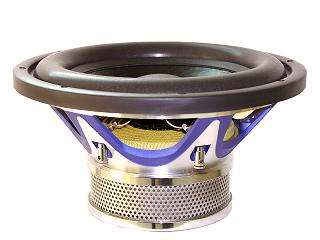recommended cabinet 1:
closed cabinet with 12,7 L volume
from 72/46 Hz (-3dB/-8dB)

recommended cabinet 2:
43 L volume bass reflex cabinet
with HP100 reflex tube, 33 cm long.
from 38/30 Hz (-3dB/-8dB).

## OmnesAudio SW 12.01 PC

order no. not-available- not available

31 cm bass driver with paper cone.

• power handling (continuous/programme) = 350/600 W
• frequency range = 32-500 Hz
• resonance frequency fs = 31,6 Hz
• impedance R = 4 Ohm
• sound pressure level SPL = 89,4 dB (2,83V; 1m)
• DC resistance Re = 3,2 Ohm
• force factor BL = 15,1 N/A
• voice coil inductance L = 2,39 mH
• effective piston radiating area Sd = 479 cm2
• effective mechanical mass incl. air load mms = 126 g
• equivalent volume of compliance Vas = 64,3 l
• total Q factor Qts = 0,34 (Qms=9,49, Qes=0,35)
• voice coil diameter = 63 mm
• maximum peak linear excursion vibration xlin = +/- 12 mm
• mounting diameter d = 285 mm
• overall diameter d = 325 mm
• mounting depth (not countersunk) t = 155 mm
PDF data sheetrecommended cabinet 1:
closed cabinet with 11,5 L volume
from 80/50 Hz (-3dB/-8dB)

recommended cabinet 2:
46 L volume bass reflex cabinet
with HP70 reflex tube, 9 cm long.
from 36/31 Hz (-3dB/-8dB).

## OmnesAudio MW 5W

order no. BP-mw5w- not available

14 cm bass-midrange driver with paper cone. To avoid compression effects the driver possesses a pole piece hole. The spider is rear ventilated for better cooling.

• frequency range = 60-6000 Hz
• resonance frequency fs = 56 Hz
• impedance R = 6 Ohm
• sound pressure level SPL = 88 dB (2,83V; 1m)
• DC resistance Re = 5,24 Ohm
• force factor BL = 4,53 N/A
• effective piston radiating area Sd = 95 cm2
• effective mechanical mass incl. air load mms = 7,2 g
• equivalent volume of compliance Vas = 14 l
• total Q factor Qts = 0,56 (Qms=3,85, Qes=0,65)
• voice coil diameter = 25,55 mm
• mounting diameter d = 124 mm
• overall diameter d = 150 mm
• mounting depth (not countersunk) t = 71 mm
PDF data sheetopen a bigger photo

recommended cabinet 1:
closed cabinet with 7,7 L volume
from 88/56 Hz (-3dB/-8dB)

recommended cabinet 2:
29 L volume bass reflex cabinet
with HP70 reflex tube, 13 cm long.
from 44/35 Hz (-3dB/-8dB).

## OmnesAudio MW 6B

order no. BP-mw6b- not available

16 cm bass-midrange driver with paper cone. To avoid compression effects the driver possesses a pole piece hole. The spider is rear ventilated for better cooling.

• frequency range = 50-5000 Hz
• resonance frequency fs = 44 Hz
• impedance R = 8 Ohm
• sound pressure level SPL = 87 dB (2,83V; 1m)
• DC resistance Re = 6,3 Ohm
• force factor BL = 7 N/A
• effective piston radiating area Sd = 135 cm2
• effective mechanical mass incl. air load mms = 12 g
• equivalent volume of compliance Vas = 28 l
• total Q factor Qts = 0,4 (Qms=7,4, Qes=0,42)
• voice coil diameter = 32,5 mm
• mounting diameter d = 147 mm
• overall diameter d = 180 mm
• mounting depth (not countersunk) t = 78 mm
PDF data sheetrecommended cabinet 1:
closed cabinet with 5,4 L volume
from 87/55 Hz (-3dB/-8dB)

recommended cabinet 2:
17 L volume bass reflex cabinet
with HP70 reflex tube, 27 cm long.
from 47/36 Hz (-3dB/-8dB).

## OmnesAudio MW 8W

order no. BP-mw8w- not available

20 cm bass-midrange driver with paper cone. To avoid compression effects the driver possesses a pole piece hole. The spider is rear ventilated for better cooling.

• frequency range = 35-3000 Hz
• resonance frequency fs = 35 Hz
• impedance R = 8 Ohm
• sound pressure level SPL = 90 dB (2,83V; 1m)
• DC resistance Re = 6 Ohm
• force factor BL = 11,83 N/A
• effective piston radiating area Sd = 214 cm2
• effective mechanical mass incl. air load mms = 39 g
• equivalent volume of compliance Vas = 33,8 l
• total Q factor Qts = 0,31 (Qms=6,77, Qes=0,33)
• voice coil diameter = 38,55 mm
• mounting diameter d = 184 mm
• overall diameter d = 220 mm
• mounting depth (not countersunk) t = 100 mm
PDF data sheet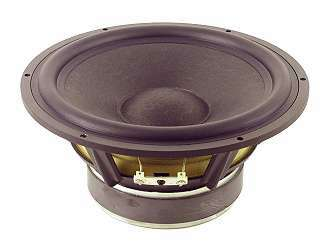## OmnesAudio MW 8.01 PC-S

order no. not-available- not available

19 cm bass driver with paper cone.

• power handling (continuous/programme) = 100/130 W
• frequency range = 30-3000 Hz
• resonance frequency fs = 37,6 Hz
• impedance R = 8 Ohm
• sound pressure level SPL = 86 dB (2,83V; 1m)
• DC resistance Re = 7,2 Ohm
• force factor BL = 7,2 N/A
• voice coil inductance L = 0,5 mH
• effective piston radiating area Sd = 214 cm2
• effective mechanical mass incl. air load mms = 28,28 g
• equivalent volume of compliance Vas = 40,7 l
• total Q factor Qts = 0,68 (Qms=2,43, Qes=0,94)
• voice coil diameter = 38 mm
• maximum peak linear excursion vibration xlin = +/- 5 mm
• mounting diameter d = 183 mm
• overall diameter d = 203 mm
• mounting depth (not countersunk) t = 86 mm
PDF data sheetrecommended cabinet 1:
closed cabinet with 12,8 L volume
from 69/43 Hz (-3dB/-8dB)

recommended cabinet 2:
52 L volume bass reflex cabinet
with HP70 reflex tube, 11 cm long.
from 31/27 Hz (-3dB/-8dB).

## OmnesAudio MW 7.01 PC-S

order no. not-available- not available

16 cm bass driver with paper cone.

• power handling (continuous/programme) = 80/100 W
• frequency range = 40-4000 Hz
• resonance frequency fs = 46 Hz
• impedance R = 8 Ohm
• sound pressure level SPL = 88 dB (2,83V; 1m)
• DC resistance Re = 7,1 Ohm
• force factor BL = 6,48 N/A
• voice coil inductance L = 1,02 mH
• effective piston radiating area Sd = 138,9 cm2
• effective mechanical mass incl. air load mms = 16,8 g
• equivalent volume of compliance Vas = 19,08 l
• total Q factor Qts = 0,53 (Qms=1,79, Qes=0,76)
• voice coil diameter = 38 mm
• maximum peak linear excursion vibration xlin = +/- 5 mm
• mounting diameter d = 147 mm
• overall diameter d = 167 mm
• mounting depth (not countersunk) t = 93 mm
PDF data sheetrecommended cabinet 1:
closed cabinet with 2,5 L volume
from 102/64 Hz (-3dB/-8dB)

recommended cabinet 2:
8,5 L volume bass reflex cabinet
with HP50 reflex tube, 20 cm long.
from 53/42 Hz (-3dB/-8dB).

## OmnesAudio MW 6W

order no. not-available- not available

16 cm bass-midrange driver with paper cone. To avoid compression effects the driver possesses a pole piece hole. The spider is rear ventilated for better cooling.

• resonance frequency fs = 44,57 Hz
• impedance R = 8 Ohm
• DC resistance Re = 6,3 Ohm
• effective piston radiating area Sd = 145 cm2
• effective mechanical mass incl. air load mms = 12,42 g
• equivalent volume of compliance Vas = 12,73 l
• total Q factor Qts = 0,35 (Qms=7,38, Qes=0,37)
• voice coil diameter = 32,5 mm
• mounting diameter d = 148 mm
• overall diameter d = 180 mm
• mounting depth (not countersunk) t = 84 mm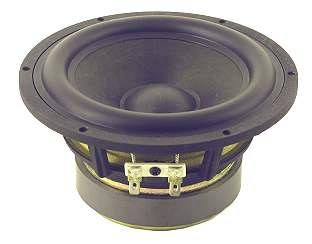## OmnesAudio MW 5.01 PC-S

order no. not-available- not available

13 cm bass driver with paper cone.

• power handling (continuous/programme) = 60/80 W
• frequency range = 40-5000 Hz
• resonance frequency fs = 54,16 Hz
• impedance R = 8 Ohm
• sound pressure level SPL = 85 dB (2,83V; 1m)
• DC resistance Re = 7,2 Ohm
• force factor BL = 6,24 N/A
• voice coil inductance L = 0,77 mH
• effective piston radiating area Sd = 18,92 cm2
• effective mechanical mass incl. air load mms = 7,58 g
• equivalent volume of compliance Vas = 10,4 l
• total Q factor Qts = 0,68 (Qms=3,87, Qes=0,82)
• voice coil diameter = 25 mm
• maximum peak linear excursion vibration xlin = +/- 3,5 mm
• mounting diameter d = 122 mm
• overall diameter d = 142 mm
• mounting depth (not countersunk) t = 97 mm
PDF data sheet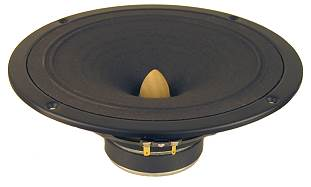## OmnesAudio L8

order no. not-available- not available

21 cm full range driver with paper cone.

• power handling (continuous/programme) = 30/60 W
• frequency range = 100-17000 Hz
• resonance frequency fs = 95 Hz
• impedance R = 8 Ohm
• sound pressure level SPL = 96 dB (2,83V; 1m)
• DC resistance Re = 6,2 Ohm
• force factor BL = 5,69 N/A
• voice coil inductance L = 0,42 mH
• effective piston radiating area Sd = 219 cm2
• effective mechanical mass incl. air load mms = 9,18 g
• equivalent volume of compliance Vas = 15 l
• total Q factor Qts = 1,04 (Qms=7,1, Qes=1,22)
• mounting diameter d = 190 mm
• overall diameter d = 230 mm
• mounting depth (not countersunk) t = 87 mm
PDF data sheet## OmnesAudio MT 2.01

order no. not-available- not available

ribbon tweeter.

• frequency range = 1200-30000 Hz
• resonance frequency fs = 1500 Hz
• impedance R = 8 Ohm
• sound pressure level SPL = 92 dB (2,83V; 1m)
• DC resistance Re = 7,6 Ohm
• mounting depth (not countersunk) t = 22 mm
PDF data sheet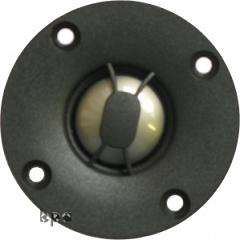## OmnesAudio T 25 Ti

order no. not-available- not available

.

• resonance frequency fs = 1700 Hz
• impedance R = 4 Ohm
• sound pressure level SPL = 93 dB (2,83V; 1m)## OmnesAudio T 2.01

order no. not-available- not available

25 mm tweeter with silk cone.

• frequency range = 2500-20000 Hz
• resonance frequency fs = 1500 Hz
• impedance R = 8 Ohm
• sound pressure level SPL = 90 dB (2,83V; 1m)
• voice coil diameter = 25 mm
• mounting diameter d = 72 mm
• overall diameter d = 99,5 mm
• mounting depth (not countersunk) t = 35 mm
PDF data sheet

The cabinet volume calculations are executed considering an external resistance of 0.3 Ohm. We are using, if no other data is known or available, the information supplied by the manufacturer.

Please note: the frequency response doesn't say much about the cut-off frequency inside the cabinet! Therefore, we mostly give you 2 examples with dimensioning considerations and an indication, how low the loudspeaker will reproduce.

Home

© Copyright 2013 Iris Strassacker; All rights reserved. - errors and omissions excepted